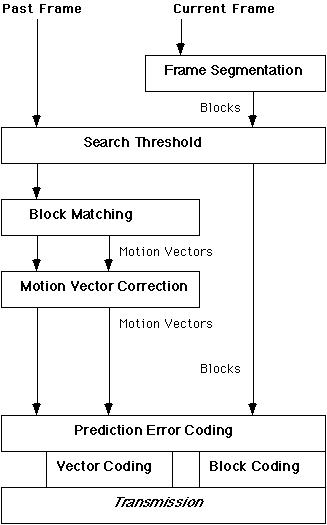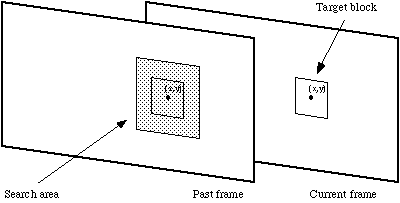## Motion Compensated Video Compression OverviewFlow of information through the motion compensation process.

As described in the section on Interframe Compression techniques, block based motion compensation uses blocks from a past frame to construct a replica of the current frame. The past frame is a frame that has already been transmitted to the receiver. For each block in the current frame a matching block is found in the past frame and if suitable, its motion vector is substituted for the block during transmission. Depending on the search threshold some blocks will be transmitted in their entirety rather than substituted by motion vectors. The problem of finding the most suitable block in the past frame is known as the block matching problem.

Block based motion compensated video compression takes place in a number of distinct stages. The flow chart above illustrates how the output from the earlier processes form the input to later processes. Consequently choices made at early stages can have an impact of the effectiveness of later stages. To fully understand the issues involved with this type of video compression it is necessary to examine each of the stages in detail. These stages can be described as:
• Frame Segmentation
• Search Threshold [»]
• Block Matching [»]
• Motion Vector Correction [»]
• Vector Coding [»]
• Prediction Error Coding [»]

### Frame Segmentation

The current frame of video to be compresfsed is divided into equal sized non-overlapping rectangular blocks. Ideally the frame dimensions are multiples of the block size and square blocks are most common. Chan et al. used rectangular blocks of 16 x 8 pixels, claiming that blocks of this shape exploit the fact that motion within image sequences is more often in the horizontal direction than the vertical [Chan94].

Block size affects the performance of compression techniques. The larger the block size, the fewer the number of blocks, and hence fewer motion vectors need to be transmitted. However, borders of moving objects do not normally coincide with the borders of blocks and so larger blocks require more correction data to be transmitted [Lin95]. Small blocks result in a greater number of motion vectors, but each matching block is more likely to closely match its target and so less correction data is required. Lallaret et al. found that if the block size is too small then the compression system will be very sensitive to noise [Lall91]. Thus block size represents a trade off between minimising the number of motion vectors and maximising the quality of the matching blocks. The relationship between block size, image quality, and compression ratio has been the subject of much research and is well understood [Nels93].

For architectural reasons block sizes of integer powers of 2 are preferred and so block sizes of 8 and 16 pixels predominate. Both the MPEG and H.261 video compression standards use blocks of 16x16 pixels.

### Search Threshold

If the difference between the target block and the candidate block at the same position in the past frame is below some threshold then it is assumed that no motion has taken place and a zero vector is returned. Thus the expense of a search is avoided. Most video codecs employ a threshold in order to determine if the computational effort of a search is warranted.

### Block Matching

Block matching is the most time consuming part of the encoding process. During block matching each target block of the current frame is compared with a past frame in order to find a matching block. When the current frame is reconstructed by the receiver this matching block is used as a substitute for the block from the current frame.

Block matching takes place only on the luminance component of frames. The colour components of the blocks are included when coding the frame but they are not usually used when evaluating the appropriateness of potential substitutes or candidate blocks.Corresponding blocks from a current and past frame, and the search area in the past frame.

The search can be carried out on all of the past frame, but is usually restricted to a smaller search area centred around the position of the target block in the current frame (see above figure). This practice places an upper limit, known as the maximum displacement, on how far objects can move between frames, if they are to be coded effectively. The maximum displacement is specified as the maximum number of pixels in the horizontal and vertical directions that a candidate block can be from the position of the target block in the original frame.

The quality of the match can often be improved by interpolating pixels in the search area, effectively increasing the resolution within the search area by allowing hypothetical candidate blocks with fractional displacements.

The search area need not be square. Because motion is more likely in the horizontal direction than vertical [Chan94], rectangular search areas are popular. The CLM460x MPEG video encoder (CCube 1992), for example, uses displacements of -106 to +99.5 pixels in the horizontal direction, and -58 to +51.5 pixels in the vertical. The half pixel accuracy is the result of the matching including interpolated pixels. The cheaper CLM4500, on the other hand, uses ±48 pixels in the horizontal direction, and ±24 in the vertical, again with half pixel accuracy.

If the block size is b and the maximum displacements in the horizontal and vertical directions are dx and dy respectively, then the search area will be of size (2dx + b)(2dy + b). Excluding sub-pixel accuracy it will contain (2dx + 1)(2dy+1) distinct, but overlapping, candidate blocks. Clearly the larger the allowable displacement the greater the probability of finding a good match. The number of candidate blocks in the search area, however, increases quadratically as the displacement increases, which can result in a large number of candidate blocks being compared to the target block. Considering every candidate block in the search area as a potential match is known as an Exhaustive Search, Brute Force Search, or Full Search

### Matching Criteria

In order for the compressed frame to look like the original, the substitute block must be as similar as possible to the one it replaces. Thus a matching criterion, or distortion function, is used to quantify the similarity between the target block and candidate blocks. If, due to a large search area, many candidate blocks are considered, then the matching criteria will be evaluated many times. Thus the choice of the matching criteria has an impact on the success of the compression. If the matching criterion is slow, for example, then the block matching will be slow. If the matching criterion results in bad matches then the quality of the compression will be adversely affected. Fortunately a number of matching criteria are suitable for use in video compression.

[The matching criteria page has more detail on this topic]

#### Sub-Optimal Block Matching Algorithms

The exhaustive search is computationally very intensive and requires the distortion function (matching criteria) to be evaluated many times for each target block to be matched. Considerable research has gone into developing block matching algorithms that find suitable matches for target blocks but require fewer evaluations. Such algorithms test only some of the candidate blocks from the search area and choose a match from this subset of blocks. Hence they are known as sub-optimal algorithms. Because they do not examine all of the candidate blocks, the choice of matching block might not be as good as that chosen by an exhaustive search. The quality-cost trade-off is usually worthwhile however.

[There is a lot more information is available on block matching at this site.]

### Motion vector correction

Once the best substitute, or matching block, has been found for the target block, a motion vector is calculated. The motion vector describes the location of the matching block from the past frame with reference to the position of the target block in the current frame.

Motion vectors, irrespective of how they are determined, might not correspond to the actual motion in the scene. This may be due to noise, weaknesses in the matching algorithm, or local minima. The property that is exploited in spatially dependent algorithms, can be utilised after the vectors have been calculated in an attempt to correct them. Smoothing techniques can be applied to the motion vectors that can detect erratic vectors and suggest alternatives. The alternative motion vectors can be used in place of those suggested by the BMA. Usually the candidate blocks to which they refer are first evaluated as potential matches and the corrected motion vector used only if the block is suitable [Choi89].

Smoothing motion vectors, however, can add considerable complexity to a video compression algorithm and should only be used where the benefits outweigh these costs. If frames are going to be interpolated by the receiver then motion vector correction is likely to be worthwhile. Smoothing can also reduce the amount of data required to transmit the motion vector information, because this information is subsequently compressed and smooth vectors can be compressed more efficiently.

Vector smoothing causes problems of its own. Smoothing can cause small objects to be coded badly because their motion vectors might be considered erroneous when they are in fact correct. Smoothing such motion vectors can adversely affect the quality of the compressed image. Stiller found that averaging schmes blurred sharp discontinuties in image sequences. Because these discontinuities might be the result of object boundaries they should be preserved [Stil90]. To correct these problems Kim & Park developed a sophisticated correction process that yielded better results than other methods [Kim93]. Lallauret & Barba devised a technique that reconsidered every motion vector that did not concur with its immediate neighbours to the left and above [Lall91].

### Vector coding

Once determined, motion vectors must be assigned bit sequences to represent them. Because so much of the compressed data will consist of motion vectors, the efficiency with which they are coded has a great impact on the compression ratio [Jang91]. In fact up to 40% of the bits transmitted by a codec might be taken up with motion vector data [Choi89]. Fortunately, the high correlation between motion vectors and their non-uniform distribution makes them suitable for further compression. This compression must be lossless.

Efficient coding of motion vectors is a subject of research in its own right and many authors have offered suggestions on which techniques work best. Any one of the lossless general purpose compression algorithms are suitable for coding vectors. Akansu et al investigated the relative efficiency of Arithmetic, Adaptive Huffman, and Lewpel-Ziv Coding [Akan89]. They found that the arithmetic and Huffman techniques performed best and that adaptive techniques using short term statistics performed better than those using long term statistics. The ISO/IEC video compression standard known as MPEG specifies variable length codes to be used for motion vectors. The zero vector, for example, has a short code, because it is the most frequently occuring.

Lallauret & Barba tested two methods of coding motion vectors. The first was a predictive method where a prediction of the motion vector was calculated based on its predecessors in the same row and column. The prediction errors were then Huffman coded. The second technique grouped the motion vectors into blocks. If all the vectors in a block were the same, then only one was transmitted. Blocks that did not contain a homogenous set of vectors were labelled and the vectors described as per as their first method [Lall91].

### Prediction Error coding

Although the battery of techniques described thus far can code video very successfully, they rarely generate perfect replicas of the original frames. Thus the difference between a predicted frame and the original uncompressed frame might be coded. Generally this is applied on a block by block basis and only where portions of the coded frame are significantly different from the original. Transform coding is most frequently used to achieve this and completely lossless coding is rarely a goal.